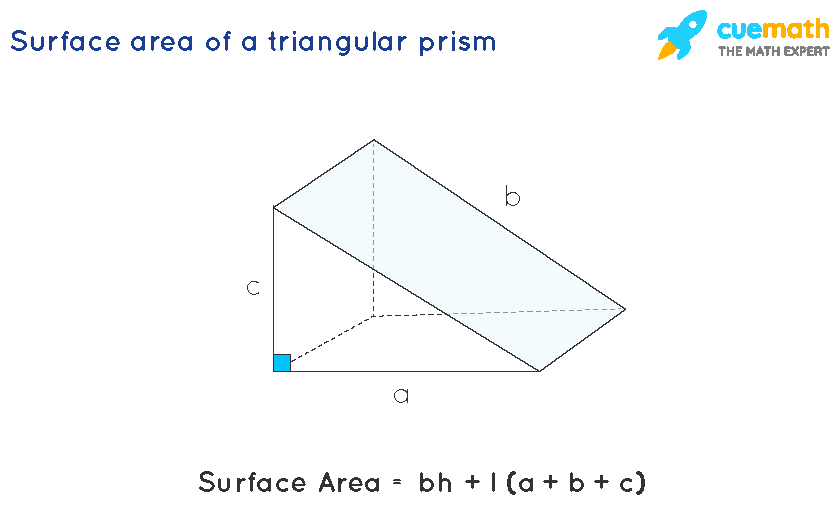# The formula to find the surface area of a triangular prism?

In mathematics, a triangular prism is a polyhedron that is made up of two triangular bases and three rectangular sides. Surface area can be defined as the total area that the surface of a particular object occupies

## Answer: The formula to find the surface area of a triangular prism is bh + l (a+b+c).

Let's understand the formula in detail.

Explanation:

The surface area of a triangular prism can be calculated by adding up the area of all rectangular and triangular faces.

Let's solve this question with a figure given below:The surface area of the triangular Prism = (2 × base area of a triangle) + (height of the prism × perimeter of the base)

The surface area of the triangular Prism = [2 ×(1/2)×bh + l×(a+b+c)]

Therefore, the formula surface area of a triangular prism = bh + l (a+b+c)

where,

'b' is the base of the base triangle.

'h' is the height of the base triangle

l = height of the prism or distance between triangular bases

a, b, c = sides of the triangle

%--split--%

## Text Solution

Learn from the best math teachers and top your exams

• Live one on one classroom and doubt clearing
• Practice worksheets in and after class for conceptual clarity
• Personalized curriculum to keep up with school